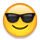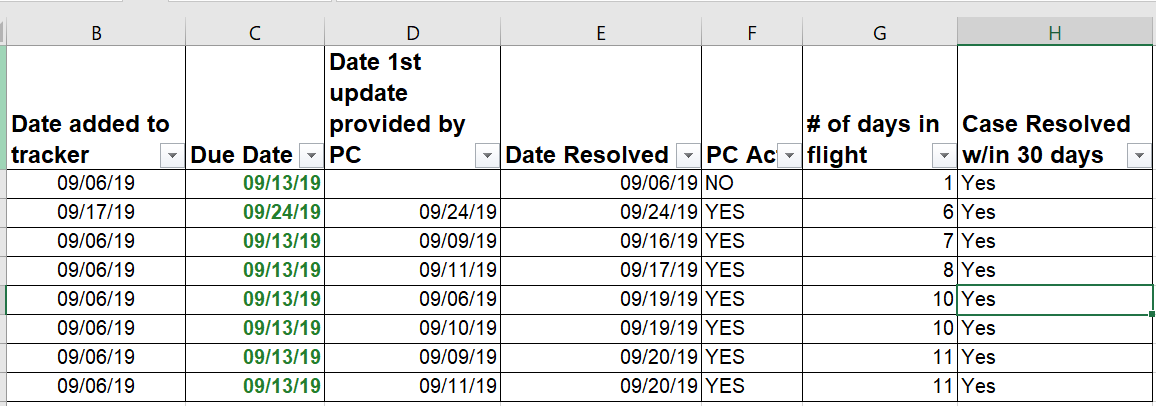# Function/Formula help to input Yes/No values as well as days outstanding based on two different scen

Hello,

Can you please help me figure out formula's for the follow scenarios? I have attached a copy of my smart sheet that i'm needing to apply the formulas to.

#1) PC Action w/in 5 days (Column F):  If the date in column D (Date 1st update provided by PC) is less than or equal to the date in column C(Due Date) then YES would show in column F, if the date in column D is later than it would show NO.

#2) Case resolved w/in 30 days (Column H) : If the date resolved (Column E) is 30 days or less from the date added to tracker (Column= YES; If the date in column E is greater than 30 days from the date added to the tracker  = NO

#3)  # of days in flight (column G) -  I am using the below formula to show number of days from the time the item was added to smart sheet to the time it took to resolve. I need help figuring out what i need to add to the formula for this show # of days from date added to tracker to today if Date Resolved field (Column E) is blank.

=NETWORKDAYS([Date added to tracker]460, [Date Resolved]460)

Any guidance you could provide would be greatly appreciated!

Thanks so much!

TiffanyTags:

• Hello Tifair,

Thank you for reaching out within the Community.

I have performed some testing and have created a formula for each example. Please note that these are examples and will require modification to work within your sheet.

1) =IF([Date 1st]@row<= [Due Date]@row, "Yes", "No")

2) =IF([Date Resolved]@row - [Date added to Tracker]@row <= 30, "Yes", "No")

3) =IF(ISBLANK([Date Resolved]@row), NETDAYS(TODAY(), [Date added to Tracker]@row), NETDAYS([Date added to Tracker]@row, [Date Resolved]@row) - 1)

Here are the articles on the functions I used to create each of the Formulas:

If you face any issues or have any further questions, please reach out

Kindest Regards

Sean

## Help Article Resources

Want to practice working with formulas directly in Smartsheet?

Check out the Formula Handbook template!